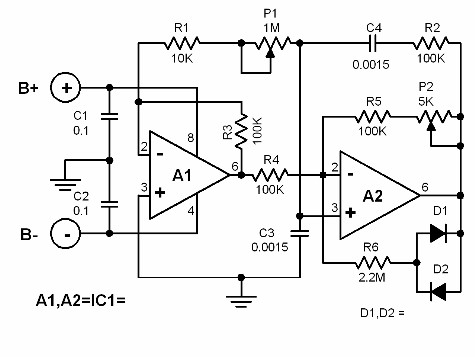# Wienbridge Oscillator Circuit Diagrams

Wienbridge Oscillator Circuit Some electronic hobbyists are not interested in wienbridge oscillators because of the difficulty in tuning it to the desired frequency. In order to change the frequency of a wienbridge, one must adjust either two resistors or two capacitors simultaneously.Wienbridge Oscillator Circuit Diagrams

### Wienbridge Oscillator Circuit

Some electronic hobbyists are not interested in wienbridge oscillators because of the difficulty in tuning it to the desired frequency.

In order to change the frequency of a wienbridge, one must adjust either two resistors or two capacitors simultaneously.

This problem, however, can be avoided by using the circuit featured here. It is still a wienbridge oscillator but its frequency can be varied through a single potentiometer.

The frequency (fo) can be found mathematically by:

fo = 1/(2pi RC SQR(a))

where R= R2= R3= R4= R5 and C= C3= C4 and a= (P1+R1)/R1. The maximum stable amplitude can be adjusted up to a level of 3.5V.

The given component values allows the oscillator to generate frequencies between 350 Hz and 3500 Hz (3.5KHz). Potentiometer P1 varies the output frequency.